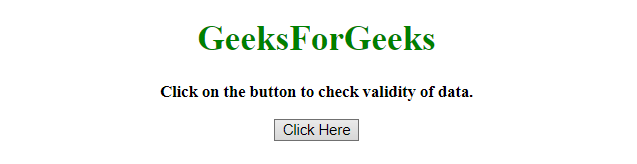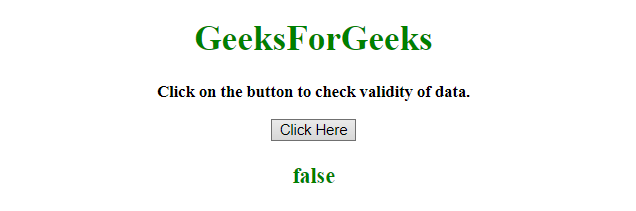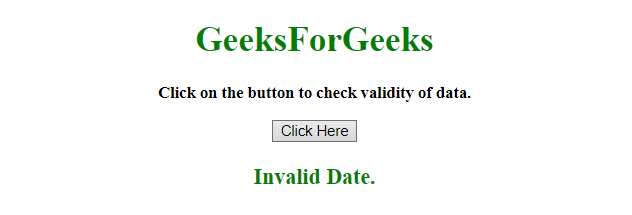# How to check a date is valid or not using JavaScript ?

• Last Updated : 21 Jul, 2021

Given a date object and the task is to check the given date is valid or not using JavaScript. There are two methods to solve this problem which are discussed below:

Approach 1:

Hey geek! The constant emerging technologies in the world of web development always keeps the excitement for this subject through the roof. But before you tackle the big projects, we suggest you start by learning the basics. Kickstart your web development journey by learning JS concepts with our JavaScript Course. Now at it's lowest price ever!

• Store the date object in a variable.
• If the date is valid then the getTime() will always be equal to itself.
• If the date is Invalid then the getTime() will return NaN which is not equal to itself.
• The isValid() function is used to check the getTime() method is equal to itself or not.

Example 1: This example implements the above approach.

 `  ` `<``html``>  ` ` `  `<``head``>  ` `    ``<``title``>  ` `        ``How to check a date is valid ` `        ``or not using JavaScript ? ` `    `` ` `  ` ` `  `<``body` `style` `= ``"text-align:center;"``>  ` `     `  `    ``<``h1` `style` `= ``"color:green;"` `>  ` `        ``GeeksForGeeks  ` `    `` ` `     `  `    ``<``p` `id` `= ``"GFG_UP"` `style` `= ` `        ``"font-size: 15px; font-weight: bold;"``> ` `    `` ` `     `  `    ``<``button` `onclick` `= ``"GFG_Fun()"``> ` `        ``Click Here ` `    `` ` `     `  `    ``<``p` `id` `= ``"GFG_DOWN"` `style` `= ` `        ``"color:green; font-size: 20px; font-weight: bold;"``> ` `    `` ` `     `  `    ``<``script``> ` `        ``var up = document.getElementById('GFG_UP');  ` `        ``var down = document.getElementById('GFG_DOWN');  ` `        ``var d = new Date("2012/2/30"); ` `         `  `        ``up.innerHTML = "Click on the button to check" ` `                    ``+ " validity of data.<``br``>"; ` `         `  `        ``Date.prototype.isValid = function () { ` `             `  `            ``// If the date object is invalid it ` `            ``// will return 'NaN' on getTime()  ` `            ``// and NaN is never equal to itself. ` `            ``return this.getTime() === this.getTime(); ` `        ``}; ` `         `  `        ``function GFG_Fun() { ` `            ``down.innerHTML = d.isValid(); ` `        ``}  ` `    ``  ` `  ` ` `  ` `

Output:

• Before clicking on the button:• After clicking on the button:Approach 2:

• Store the date object into a variable d.
• Check if the variable d is created by Date object or not by using Object.prototype.toString.call(d) method.
• If the date is valid then the getTime() method will always be equal to itself.
• If the date is Invalid then the getTime() method will return NaN which is not equal to itself.
• In this example, isValid() method is checking if the getTime() is equal to itself or not.

Example 2: This example implements the above approach.

 `  ` `<``html``>  ` ` `  `<``head``>  ` `    ``<``title``>  ` `        ``How to check a date is valid ` `        ``or not using JavaScript ? ` `    `` ` `  ` ` `  `<``body` `style` `= ``"text-align:center;"``>  ` `     `  `    ``<``h1` `style` `= ``"color:green;"` `>  ` `        ``GeeksForGeeks  ` `    `` ` `     `  `    ``<``p` `id` `= ``"GFG_UP"` `style` `= ` `        ``"font-size: 15px; font-weight: bold;"``> ` `    `` ` `     `  `    ``<``button` `onclick` `= ``"GFG_Fun()"``> ` `        ``Click Here ` `    `` ` `     `  `    ``<``p` `id` `= ``"GFG_DOWN"` `style` `= ` `        ``"color:green; font-size: 20px; font-weight: bold;"``> ` `    `` ` `     `  `    ``<``script``> ` `        ``var up = document.getElementById('GFG_UP');  ` `        ``var down = document.getElementById('GFG_DOWN');  ` `        ``var d = new Date("This is not date."); ` `         `  `        ``up.innerHTML = "Click on the button to check " ` `                    ``+ "validity of data.<``br``>"; ` `         `  `        ``function GFG_Fun() { ` `         `  `            ``if (Object.prototype.toString.call(d) ` `                                    ``=== "[object Date]") ` `            ``{ ` `                ``if (isNaN(d.getTime())) {  ` `                    ``down.innerHTML = "Invalid Date."; ` `                ``} ` `                ``else { ` `                    ``down.innerHTML = "Valid Date."; ` `                ``} ` `            ``} ` `        ``}  ` `    ``  ` `  ` ` `  ` `

Output:

• Before clicking on the button:• After clicking on the button:JavaScript is best known for web page development but it is also used in a variety of non-browser environments. You can learn JavaScript from the ground up by following this JavaScript Tutorial and JavaScript Examples.

My Personal Notes arrow_drop_up
Recommended Articles
Page :